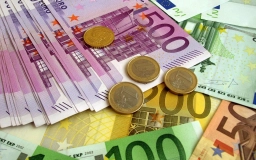# Euros 80767

Adam had 3 times more than Fero. Gabo is 11 less than Fero. They had a total of 59 euros. How much did each have?

a =  42
f =  14
g =  3

### Step-by-step explanation:

a=3f
g=f-11
a+g+f=59

a=3·f
g=f-11
a+g+f=59

a-3f = 0
f-g = 11
a+f+g = 59

Row 3 - Row 1 → Row 3
a-3f = 0
f-g = 11
4f+g = 59

Pivot: Row 2 ↔ Row 3
a-3f = 0
4f+g = 59
f-g = 11

Row 3 - 1/4 · Row 2 → Row 3
a-3f = 0
4f+g = 59
-1.25g = -3.75

g = -3.75/-1.25 = 3
f = 59-g/4 = 59-3/4 = 14
a = 0+3f = 0+3 · 14 = 42

a = 42
f = 14
g = 3

Our linear equations calculator calculates it.Did you find an error or inaccuracy? Feel free to write us. Thank you!

Tips for related online calculators
Do you have a system of equations and looking for calculator system of linear equations?